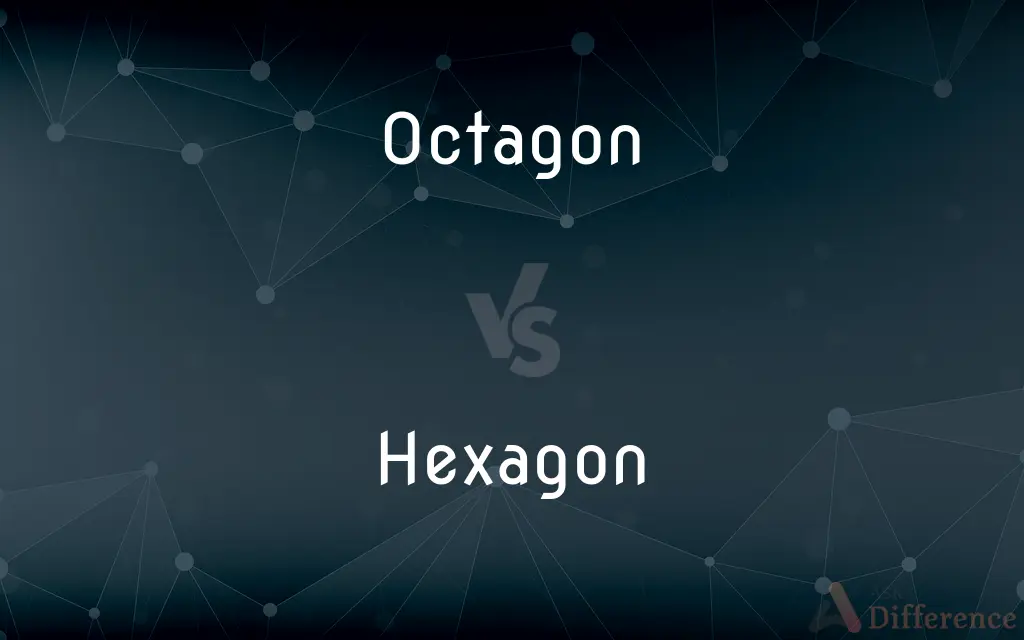# Octagon vs. Hexagon — What's the Difference?## Definitions

#### Octagon➦

In geometry, an octagon (from the Greek ὀκτάγωνον oktágōnon, "eight angles") is an eight-sided polygon or 8-gon. A regular octagon has Schläfli symbol {8} and can also be constructed as a quasiregular truncated square, t{4}, which alternates two types of edges.

#### Hexagon➦

In geometry, a hexagon (from Greek ἕξ, hex, meaning "six", and γωνία, gonía, meaning "corner, angle") is a six-sided polygon or 6-gon. The total of the internal angles of any simple (non-self-intersecting) hexagon is 720°.

#### Octagon➦

A plane figure with eight straight sides and eight angles.

#### Hexagon➦

A plane figure with six straight sides and angles.

#### Octagon➦

A polygon with eight sides and eight angles.

#### Hexagon➦

A polygon with six sides and six angles.

#### Octagon➦

A polygon with eight sides and eight angles.

#### Hexagon➦

(geometry) A polygon with six sides and six angles.

#### Octagon➦

(martial arts) Often in the form Octagon: the arena for mixed martial arts.

#### Hexagon➦

A plane figure of six angles.

#### Octagon➦

A plane figure of eight sides and eight angles.

#### Hexagon➦

A six-sided polygon